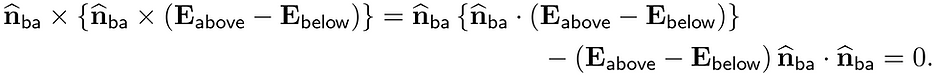## Boundary condition for tangential component of E (electrostatics case)

Here, we derive the boundary condition for the tangential component of E in a more formal way than in Griffiths, which will be particularly useful in deriving similar conditions for the magnetostatics (Ch. 5.4.2) and electrodynamics cases (Ch. 7.3.6).

Consider a figure below showing a region around a boundary with surface charge density σ(r).For a box whose top and bottom surfaces are above and below the boundary and whose area and height approach zero, we apply the following identity (Problem 1.61b in Griffiths) with v = E:For the left hand side,For the right hand side,Equating the left and right sides,